# Vibrationdata Unit 3 Sine Sweep Vibration 1 Sine

• Slides: 30
Download presentation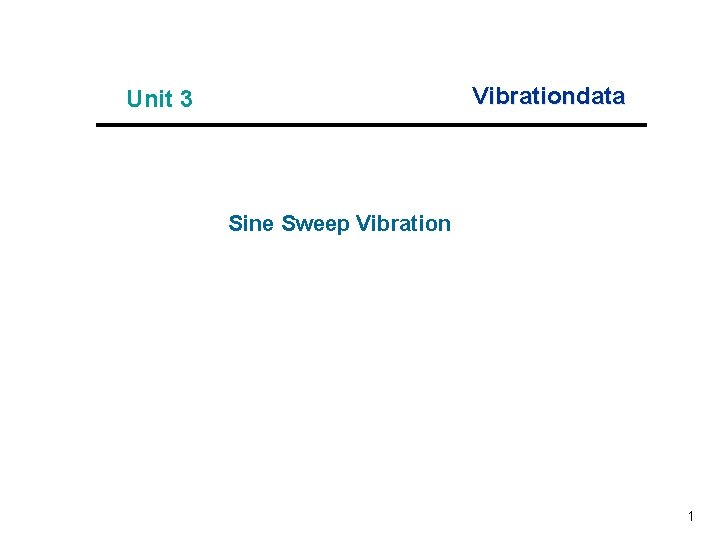Vibrationdata Unit 3 Sine Sweep Vibration 1Sine Sweep Testing Purposes Vibrationdata Sine Sweep Testing of Components and Systems n n Identify natural frequencies and amplification factors or damping ratios Perform sine sweep before and after random vibration test to determine if any parts loosened, etc. n Check for linearity of stiffness and damping n Workmanship screen for defective parts and solder joints n Represent an actual environment such as a rocket motor oscillation n NASA/GSFC typically uses sine sweep vibration for spacecraft testing 2Vibrationdata 3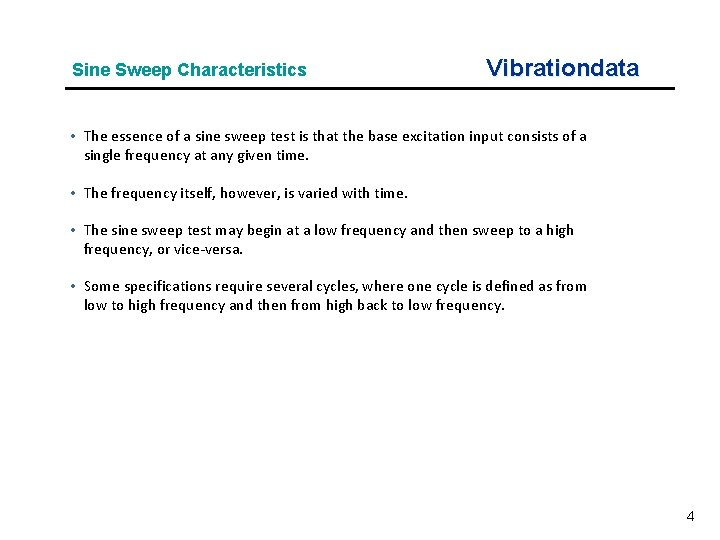Sine Sweep Characteristics Vibrationdata • The essence of a sine sweep test is that the base excitation input consists of a single frequency at any given time. • The frequency itself, however, is varied with time. • The sine sweep test may begin at a low frequency and then sweep to a high frequency, or vice-versa. • Some specifications require several cycles, where one cycle is defined as from low to high frequency and then from high back to low frequency. 4Sine Sweep Rate Vibrationdata • The specification might require either a linear or a logarithmic sweep rate. • The sweep will spend greater time at the lower frequency end if the sweep is logarithmic. • The example in the previous figure had a logarithmic sweep rate. • The amplitude in the previous is constant. • Nevertheless, the specification might require that the amplitude vary with frequency. 5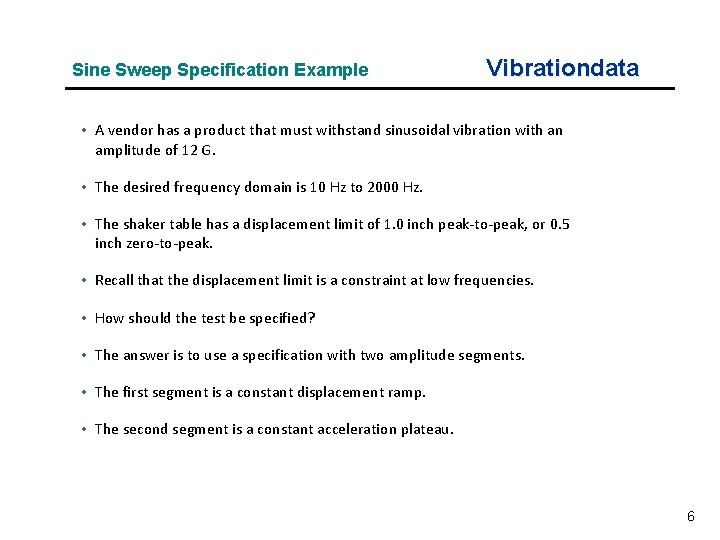Sine Sweep Specification Example Vibrationdata • A vendor has a product that must withstand sinusoidal vibration with an amplitude of 12 G. • The desired frequency domain is 10 Hz to 2000 Hz. • The shaker table has a displacement limit of 1. 0 inch peak-to-peak, or 0. 5 inch zero-to-peak. • Recall that the displacement limit is a constraint at low frequencies. • How should the test be specified? • The answer is to use a specification with two amplitude segments. • The first segment is a constant displacement ramp. • The second segment is a constant acceleration plateau. 6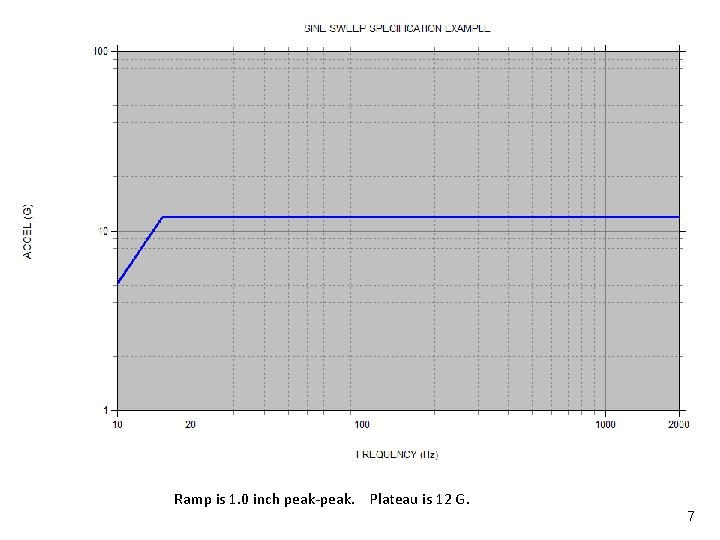Sine Amplitude Metrics Vibrationdata Ramp is 1. 0 inch peak-peak. Plateau is 12 G. 7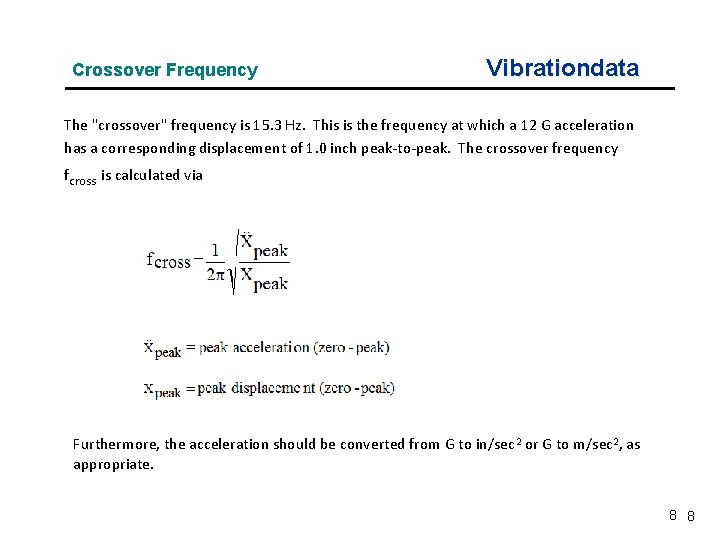Crossover Frequency Vibrationdata The "crossover" frequency is 15. 3 Hz. This is the frequency at which a 12 G acceleration has a corresponding displacement of 1. 0 inch peak-to-peak. The crossover frequency fcross is calculated via Furthermore, the acceleration should be converted from G to in/sec 2 or G to m/sec 2, as appropriate. 8 8Vibrationdata Octaves • One octave is defined as a frequency band where the upper frequency limit is equal to twice the lower frequency limit. • Thus a band from 10 Hz to 20 Hz is one octave. • Likewise, the band from 20 Hz to 40 Hz is an octave. • A concern regarding sine sweep testing is the total number of octaves. • As an example consider the following frequency sequence in Hertz. 10 - 20 - 40 - 80 -160 - 320 - 640 - 1280 – 2560 • The sequence has a total of eight octaves. 9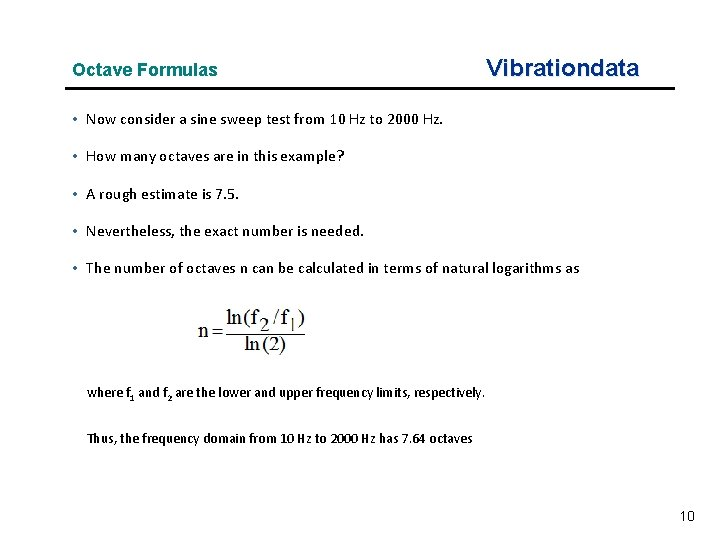Octave Formulas Vibrationdata • Now consider a sine sweep test from 10 Hz to 2000 Hz. • How many octaves are in this example? • A rough estimate is 7. 5. • Nevertheless, the exact number is needed. • The number of octaves n can be calculated in terms of natural logarithms as where f 1 and f 2 are the lower and upper frequency limits, respectively. Thus, the frequency domain from 10 Hz to 2000 Hz has 7. 64 octaves 10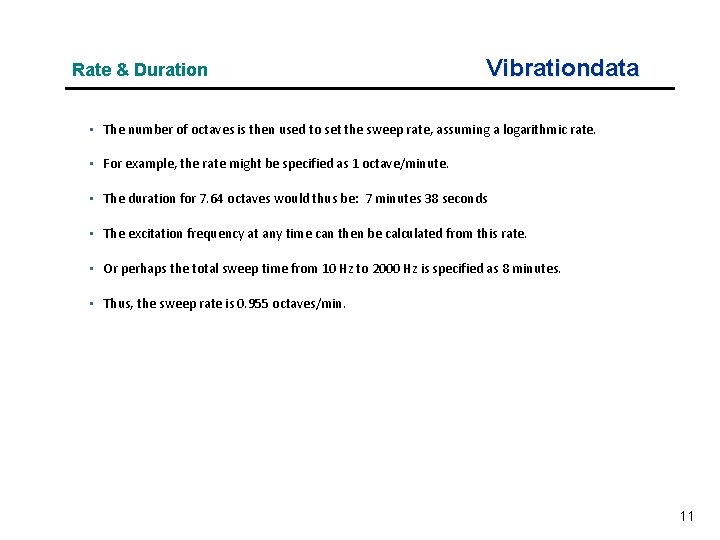Rate & Duration Vibrationdata • The number of octaves is then used to set the sweep rate, assuming a logarithmic rate. • For example, the rate might be specified as 1 octave/minute. • The duration for 7. 64 octaves would thus be: 7 minutes 38 seconds • The excitation frequency at any time can then be calculated from this rate. • Or perhaps the total sweep time from 10 Hz to 2000 Hz is specified as 8 minutes. • Thus, the sweep rate is 0. 955 octaves/min. 11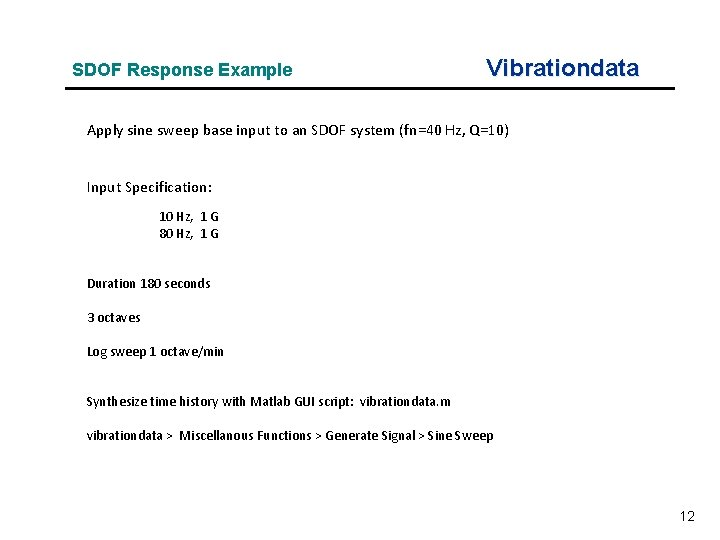SDOF Response Example Vibrationdata Apply sine sweep base input to an SDOF system (fn=40 Hz, Q=10) Input Specification: 10 Hz, 1 G 80 Hz, 1 G Duration 180 seconds 3 octaves Log sweep 1 octave/min Synthesize time history with Matlab GUI script: vibrationdata. m vibrationdata > Miscellanous Functions > Generate Signal > Sine Sweep 12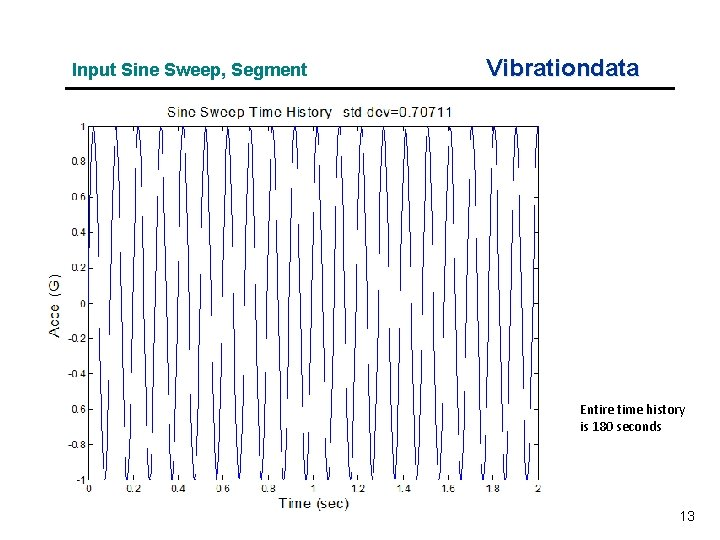Input Sine Sweep, Segment Vibrationdata Entire time history is 180 seconds 13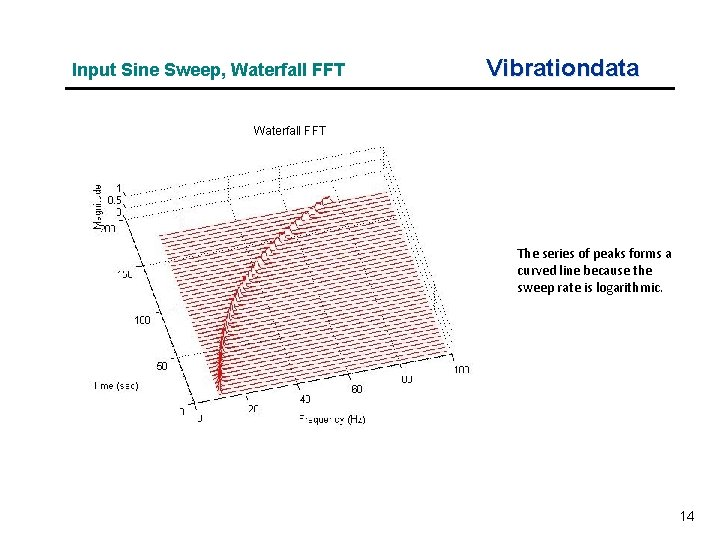Input Sine Sweep, Waterfall FFT Vibrationdata Waterfall FFT The series of peaks forms a curved line because the sweep rate is logarithmic. 14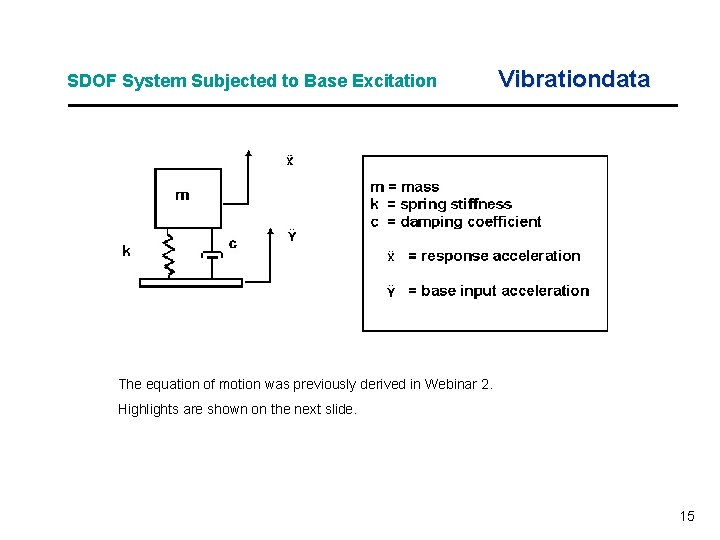SDOF System Subjected to Base Excitation Vibrationdata The equation of motion was previously derived in Webinar 2. Highlights are shown on the next slide. 15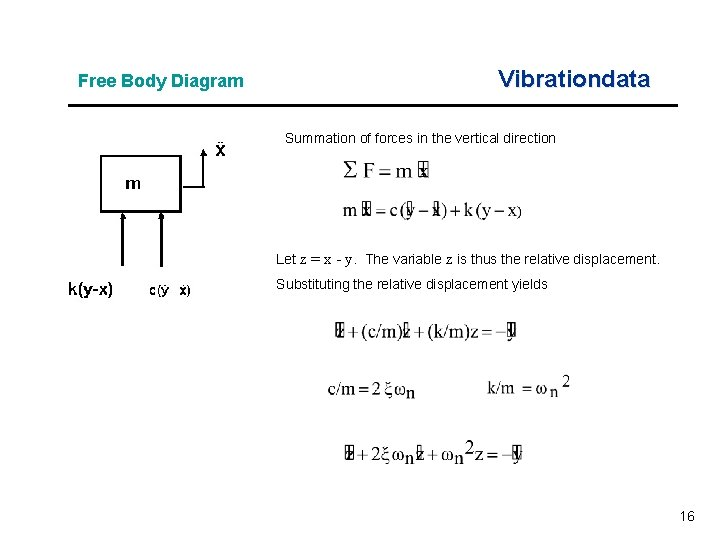Free Body Diagram Vibrationdata Summation of forces in the vertical direction Let z = x - y. The variable z is thus the relative displacement. Substituting the relative displacement yields 16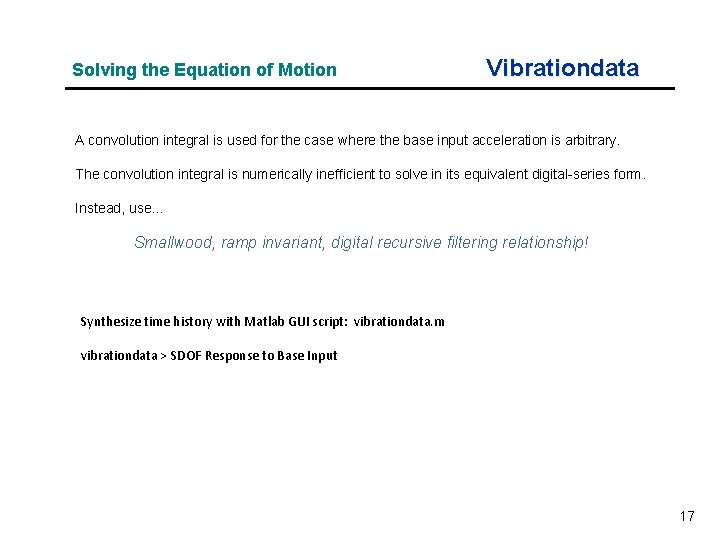Solving the Equation of Motion Vibrationdata A convolution integral is used for the case where the base input acceleration is arbitrary. The convolution integral is numerically inefficient to solve in its equivalent digital-series form. Instead, use… Smallwood, ramp invariant, digital recursive filtering relationship! Synthesize time history with Matlab GUI script: vibrationdata. m vibrationdata > SDOF Response to Base Input 17SDOF Response Vibrationdata 18Solid Rocket Pressure Oscillation Vibrationdata • Solid rocket motors may have pressure oscillations which form in the combustion chamber • Various vortex-shedding and other effects cause standing waves to form in the combustion cavity • This effect is sometimes called “Resonant Burn” or “Thrust Oscillation” • The sinusoidal oscillation frequency may sweep downward as the cavity volume increases due to the conversion of propellant to exhaust gas 19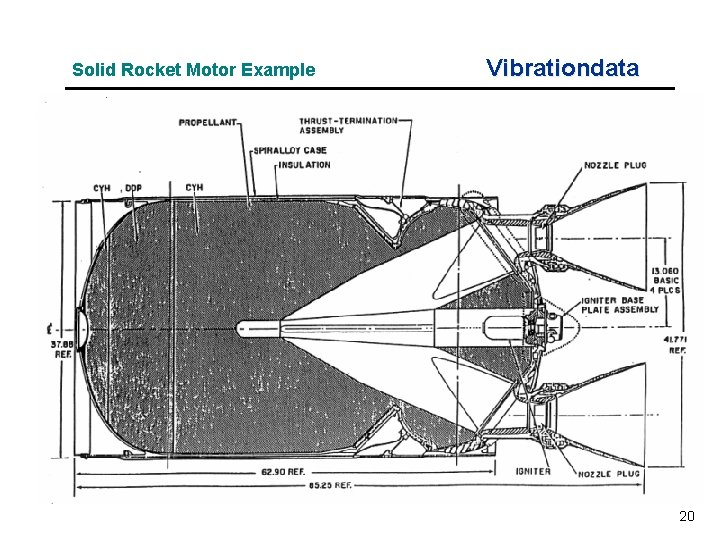Solid Rocket Motor Example Vibrationdata 20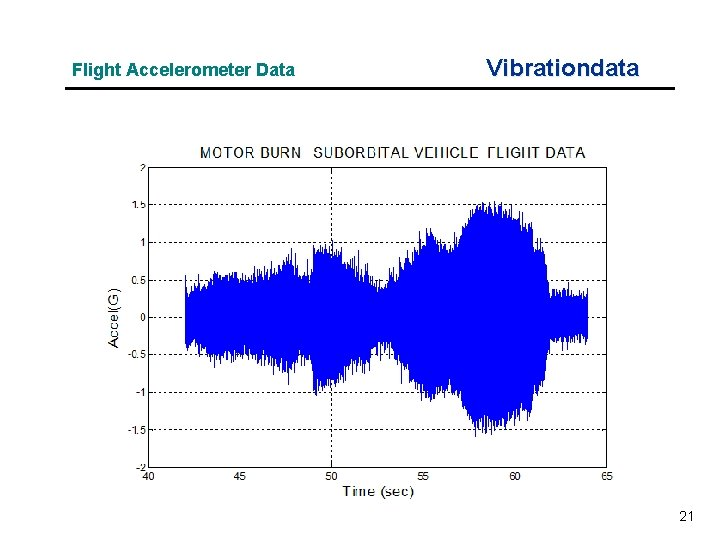Flight Accelerometer Data Vibrationdata 21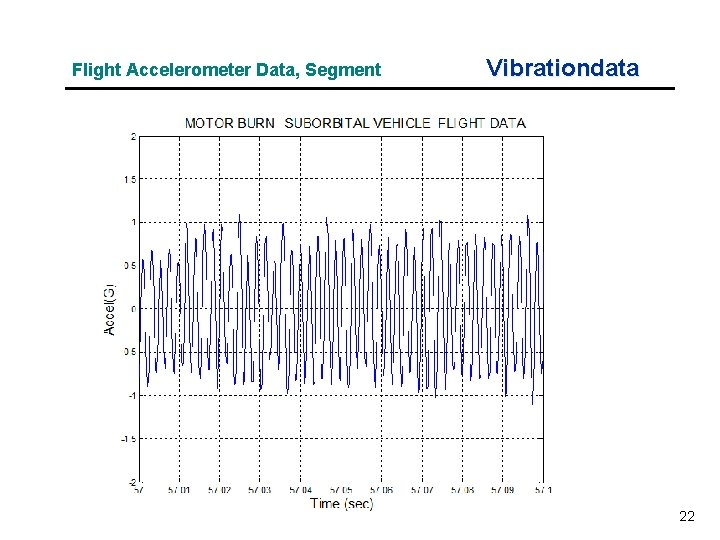Flight Accelerometer Data, Segment Vibrationdata 22Time-Varying Statistics Vibrationdata • Calculate statistics for each consecutive 0. 5 -second segment • Use Matlab GUI script: vibrationdata. m Vibrationdata > Time-Varying Freq & Amp 232425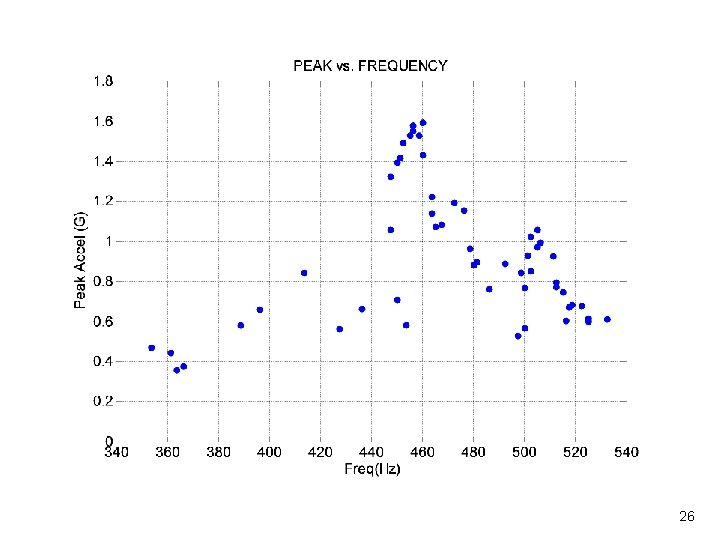26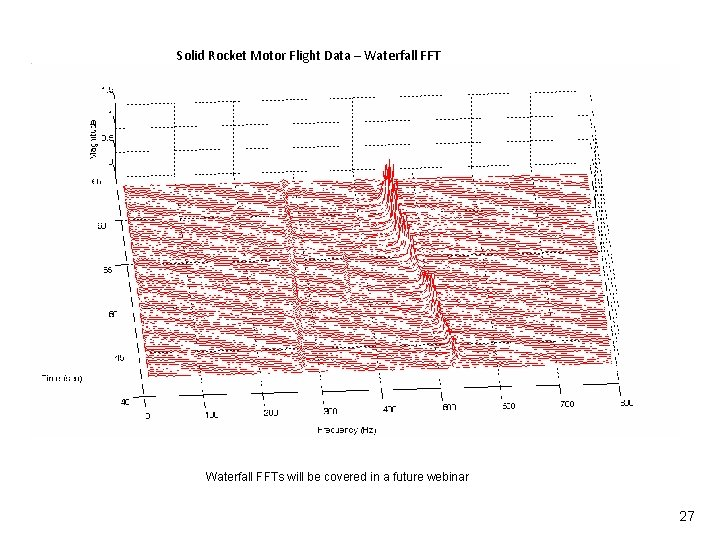Solid Rocket Motor Flight Data – Waterfall FFTs will be covered in a future webinar 27Exercise 1 Vibrationdata n A shaker table has a displacement limit of 1. 5 inch peak-to-peak, or 0. 75 inch zero-to-peak. n An amplitude of 28 G is desired from 10 Hz to 2000 Hz. n The specification will consist of a displacement ramp and an acceleration plateau. n What should the crossover frequency be? n Use Matlab GUI script: vibrationdata. m vibrationdata > Miscellaneous Functions > Sine Sweep Parameters > Cross-over Frequency n What is the maximum acceleration at 10 Hz? vibrationdata > Miscellaneous Functions > Amplitude Conversion Utilities > Steady-State Sine Amplitude 28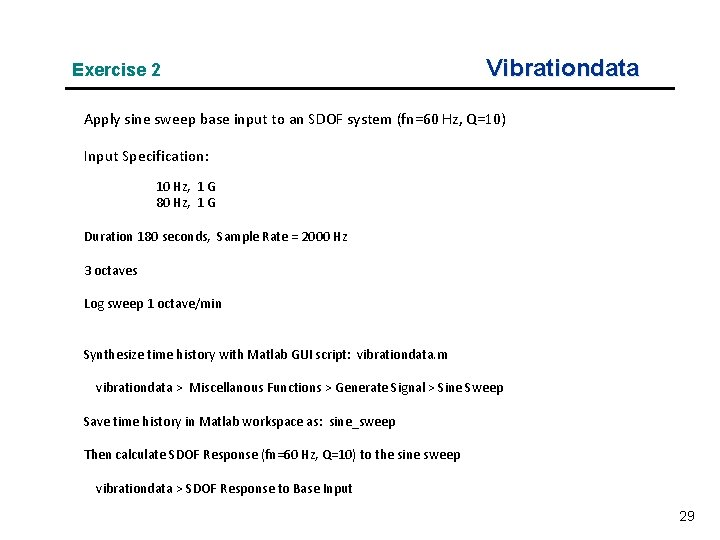Exercise 2 Vibrationdata Apply sine sweep base input to an SDOF system (fn=60 Hz, Q=10) Input Specification: 10 Hz, 1 G 80 Hz, 1 G Duration 180 seconds, Sample Rate = 2000 Hz 3 octaves Log sweep 1 octave/min Synthesize time history with Matlab GUI script: vibrationdata. m vibrationdata > Miscellanous Functions > Generate Signal > Sine Sweep Save time history in Matlab workspace as: sine_sweep Then calculate SDOF Response (fn=60 Hz, Q=10) to the sine sweep vibrationdata > SDOF Response to Base Input 29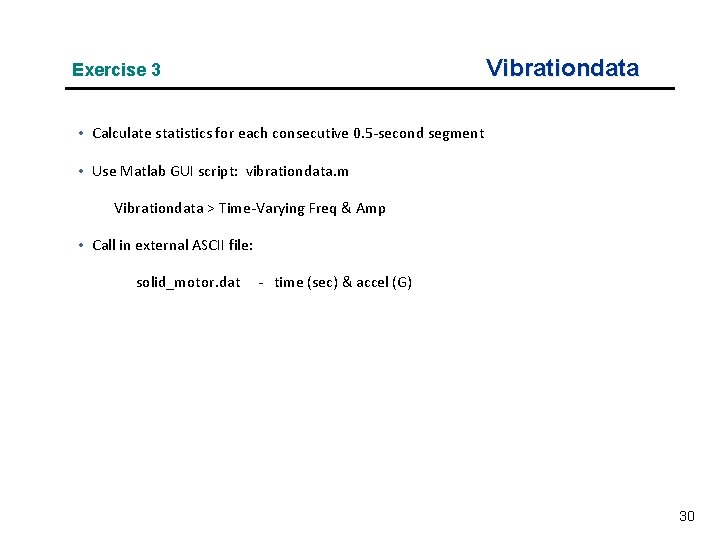Vibrationdata Exercise 3 • Calculate statistics for each consecutive 0. 5 -second segment • Use Matlab GUI script: vibrationdata. m Vibrationdata > Time-Varying Freq & Amp • Call in external ASCII file: solid_motor. dat - time (sec) & accel (G) 30